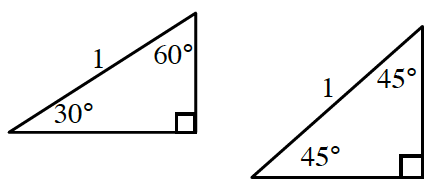### Home > CCA2 > Chapter 7 > Lesson 7.1.1 > Problem7-6

7-6.

Copy the triangles below and label the missing side lengths.Notice that these are special triangles.

For the $30^\circ-60^\circ-90^\circ\$ triangle:

short side: $\frac{1}{2}$

long side: $\frac{\sqrt{3}}{2}$